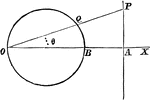### Circle

A circle and triangle situated on coordinate planes.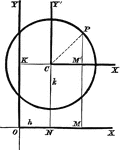### Common Form of Circle

A circle in its common, or central form. This is used to assist students in finding the equation of…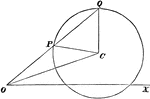### Polar Coordinates

Finding the equation of a line with polar coordinates.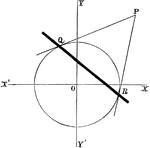### Chord Equation

Tangent lines are drawn from a given external point to a circle. The equation is found by joining their…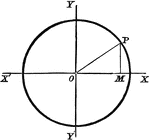### Circle Equation

Finding the equation of a circle with the origin as its center.### Normal Equation

Finding the equation of the normal at any point on a circle.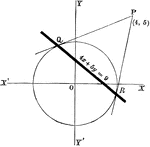### Equation Example

Example of an equation with a given external point.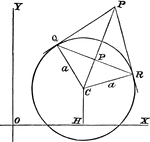### Tangent Length

Finding the length of a tangent from a given point to a circle.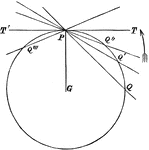### Lines

A circle with many tangent and secant lines passing through it.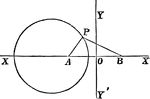### Coordinate Plane

A circle with part of a triangle inscribed in it. They are lying on a coordinate plane.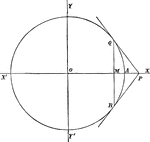### Polar of Point

Constructing the polar of a given point P with respect to a circle.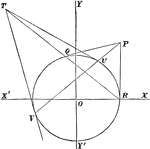### Polar Property

If the chords of a circle are drawn through a fixed point, then the points of intersection of the pairs…